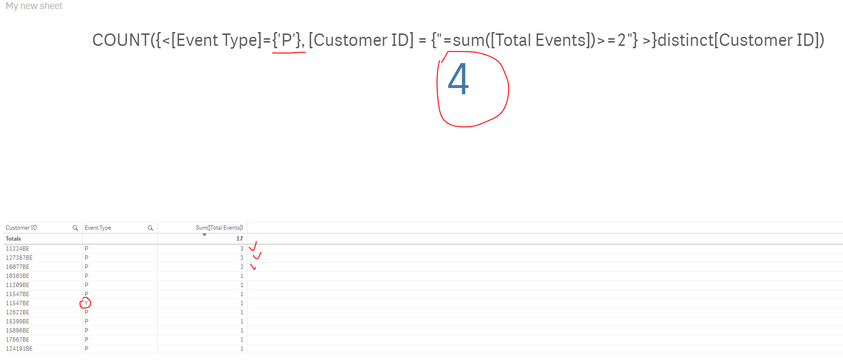# New to Qlik Sense

If you’re new to Qlik Sense, start with this Discussion Board and get up-to-speed quickly.

Announcements
QlikWorld Online 2021, May 10-12: Our Free, Virtual, Global Event REGISTER TODAY
cancel
Showing results for
Search instead for
Did you mean:Contributor III

## Count set analysis does not return expected results

Hi there , I am struggling to understand why my expression gives a wrong result.

I want to calculate customers with event type P whose total events is >= 2. The answer should be 3, but my set analysis giving me 4.

COUNT({<[Event Type]={'P'},
[Customer ID] = {"=sum([Total Events])>=2"}
>}distinct[Customer ID]

The expression seems to ignore the Event Type ='P'.

Could you please help me? I am very new to qliksense.

Thank youLabels (2)

• ### Set Analysis

1 Solution

Accepted SolutionsMVP & Luminary

Your [Event Type] condition isn't applied to the sum() and therefore there not regarded. You may change your expression like:

COUNT({<[Customer ID] = {"=sum({< [Event Type]={'P'}>} [Total Events])>=2"}
>} distinct[Customer ID]

- Marcus

16 RepliesChampion III

i think your expression to be modified like below

COUNT({<[Event Type]={'P'},
[Customer ID] = {"=count([Total Events])>=2"}
>}distinct[Customer ID])Contributor III
Author

Thanks, @Chanty4u  but that's not correct.MVP & Luminary

Your [Event Type] condition isn't applied to the sum() and therefore there not regarded. You may change your expression like:

COUNT({<[Customer ID] = {"=sum({< [Event Type]={'P'}>} [Total Events])>=2"}
>} distinct[Customer ID]

- MarcusContributor III
Author

Hi @marcus_sommer  thank you so much, it is correct now.Contributor III
Author

Hi @marcus_sommer , I have another question, for example I want to include  the last 12 month condition to the expression. Like this:

COUNT({<
[Customer ID] = {"=sum({< [Event Type]={'P'}>} [Total Events])>=2"

,Date={">=\$(=date(addmonths(Max(Date),-12),'YYYY-MM-DD'))<=\$(=date(Max(Date),'YYYY-MM-DD'))"}

>} distinct[Customer ID])

is it still correct?

Thank youContributor III
Author

The date condition does not pass to the count again 😞 could you please tell me why it is like this ? @marcus_sommerMVP & Luminary

It's the same like above - the condition must be applied to the sum() or in other words to the inner condition and not to the outer condition, maybe like this:

COUNT({<
[Customer ID] = {"=sum({< [Event Type]={'P'},
Date={">=\$(=addmonths(Max(Date),-12))<=\$(=Max(Date))"}>}
[Total Events])>=2"}
>}
distinct [Customer ID])

As far as the comparison is set to >= <= a formatting won't be needed because the evaluation happens on a numeric level - therefore date() was removed.

- MarcusContributor III
Author

Hi @marcus_sommer  the express does not return me any results.MVP & Luminary

What happens if you applies:

sum({< Date={">=\$(=addmonths(Max(Date),-12))<=\$(=Max(Date))"}>} [Total Events])

in a new table?

- Marcus